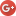## Aleksandr V. Bukalove-mail: boukalov@gmail.com
The Centre of Physical and Space Researches, International Institute of Socionics
"Physics of Consciousness and Life, Cosmology and Astrophysics" journal, issue 4, 2003 .

# The theoretical formulae for calculation of the gravitational constant and Planck units

The theoretical formulae for calculation of gravitational constant G and Planck mass, length and time are obtained. From formula it follows, that the Planck mass is only invariant mass (or rest mass) of the Planck elementary particle. The energy of this particle increases with velocity according to special relativity.
Key words: gravitational constant, Planck mass, Planck length, Planck time, mass of electron, special relativity.

The value of the gravitational constant G is known to be determined with low accuracy that is in striking contrast with other constants. It is due to the significant difficulties in experimental determination of this value. According to CODATA , the most exact experimental value makes:
Gexp = (6.673±0.010)·10-11m3·s-2·kg-1.    (1)
The theoretical formula, allowing the value G calculation with higher accuracy, until now was not known. We offer such formula.

Let us consider the correlation
mpl-12 me2/mp-12 mx,    (2)
where α=e2/Şc, mpl is Planck mass, me is mass of electron, mp is mass of proton, mx=me2/mp=278.3eV.

Proceeding from the CODATA data , α=7.297352533(27)·10-3, Ş=1.054571596(82)·10-34J·s, c=299792458 m·s-1, mp/me=1836.1526675(39)kg, me=9.10938188(72)·10-31kg we obtain
mpl=2.1756858·10-8 kg.

According to CODATA, mpl(CODATA)=2.1767(16)·10-8. The value, obtained by formula , is in a good agreement with CODATA.

From formulaand correlation (2) we obtain a formula(3)
and a value
G=6.6788915(22)·10-11m3·s-2·kg-1.    (4)

From formula (2) it follows such values of the Planck units:
mpl=2.1756858(5)·10-8kg,
lpl=1.6168407(3)·10-35m,     (5)
tpl=5.3930999(1)·10-44s.

CODATA gives such values:
lpl(CODATA)=1.6160(12)·10-35m,
tpl(CODATA)=5.3906(40)·10-44s.

From formula (2) it follows, that the Planck mass is only invariant mass (or rest mass) m0 of the Planck elementary particle, mpl=m0, εpl=mpl·c2=m0·c2, if velocity of the Planck particle is ν=0. If v / c = β ≠ 0 then.    (6)

From me2c4=εe2 - pe2c2 it follows,    (7)
Gε=GN(1–β2),,.    (8)

Then,   where,    (9)
or GN=Gt–Gx, where.    (10)

Then
Gε·Gt=Gp·Gx=GN2,    (11)
for Ş=const, c=const.(12).    (13)

The change of the values Gε, Gp,andwith velocity entails the certain changes in theories, which are based on the gravitational constant and Plank values as well as on the theory of gravity, quantum cosmology and the theory of superstrings.

References:

1. CODATA.